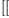login  home  contents  what's new  discussion  bug reports help  links  subscribe  changes  refresh  edit
 Topics FrontPage FriCASContributions MathMLFormat <-- You are here. The MathML package This package is now included in base system. You can get get MathML in two ways. One is to example type the command: (x+y)^2 and after that type: coerce(%)$MMLFORM or to get something that can be pasted directly into an xhtml page and displayed in Firefox type in: display(coerce(%)$MMLFORM)\$MMLFORM That gets tedious pretty quickly but there is an alternatively way. For some time mathml is added as a new output option, so issuing the command )set output mathml on will turn on mathml. The output can still be pasted into an xhtml page and viewed in Firefox however I have written a few files to enable automatic viewing in Firefox. The file serv.c is a wrapper for starting and communicating with Axiom. The trivial Makefile is for building an executable "serv". serv.c Makefile In order for this to work you need apache with PHP enabled installed. Put the following 3 files in your server directory and then open axiom.xml with Firefox, e.g. navigate to "127.0.0.1/axiom.xml" if you place the files in the root of your server directory. Axiom commands can then be typed in with the results displayed in Firefox. axiom.xml axiom.php axiom.js Here is a list of commands that format correctly, also see the pamphlet file. (x+y)^2 integrate(x^x,x) integral(x^x,x) (5 + sqrt 63 + sqrt 847)^(1/3) set1,2,3multisetx rem 5 for x in primes(2,1000)series(sin(a*x),x=0) matrixx^i + y**j for i in 1..10for j in 1..10y := operator 'y a. D(y(x,z),x,x,z,x) b. D(y x,x,2) x := series 'x a. sin(1+x) series(1/log(y),y=1) y:UTS(FLOAT,'z,0) := exp(z) a. c := continuedFraction(314159/100000) b. c := continuedFraction(314159/100000)

 Subject:   Be Bold !! ( 14 subscribers )